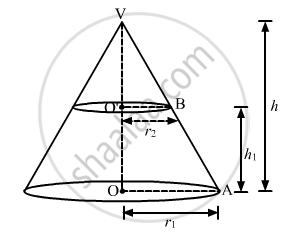Advertisement Remove all ads

# If R1 and R2 Denote the Radii of the Circular Bases of the Frustum of a Cone Such that R1 > R2, Then Write the Ratio of the Height of the Cone of Which the Frustum is a Part to - Mathematics

Answer in Brief

If r1 and r2 denote the radii of the circular bases of the frustum of a cone such that r1 > r2, then write the ratio of the height of the cone of which the frustum is a part to the height fo the frustum.

Advertisement Remove all ads

#### SolutionSince, Delta VO'B - DeltaVOA

Therefore,

In Delta VO'B - DeltaVOA

(O'B)/(OA) = (O'V)/(OV)

r_2/r_1 = (h -h_1)/(h)

r_2/r_2 = 1 - h_1/h

h_1/h = 1 - r_2/r_1

= (r_1 - r_2)/r_1

Hence,

The ratio of the height of cone of which the frustum is a part to the height fo the frustum.

$\frac{OV}{OO'} = \frac{h}{h_1} = \frac{r_1}{r_1 - r_2}$

Hence, h : h_1 = r_1 :(r_1 - r_2)

Is there an error in this question or solution?
Advertisement Remove all ads

#### APPEARS IN

RD Sharma Class 10 Maths
Chapter 14 Surface Areas and Volumes
Q 19 | Page 87
Advertisement Remove all ads

#### Video TutorialsVIEW ALL 

Advertisement Remove all ads
Share
Notifications

View all notifications

Forgot password?
Course